## Example Questions

1 3 Next →

### Example Question #21 : How To Find The Sale Price

Margaret has been given a gift card with $150 worth of credit on it. She wants to spend it at the store where her father works, because she will get a 12 1/2 % employee's family discount. Also, the sales tax at the store is 8%. The price tag on a pair of shoes that she wants to buy there says$200.00. If Margaret decides to buy the shoes and use the credit on her gift card, how much will she have to pay out of her own pocket?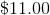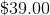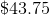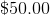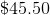Explanation:

Margaret is getting a 12 1/2 %, or 12.5 %, discount off a $200 pair of shoes; this is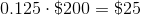Subtract this discount from the price of the shoes; Margaret will pay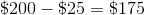, excluding sales tax. 8% of this price is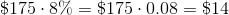, which, when added to the price, yields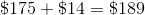. Margaret has$150 of credit on her gift card, so she will pay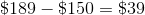out of her own pocket.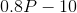dollars. Set this equal to the actual cost of the coffeemaker, $134.44: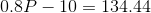Solving for: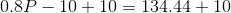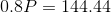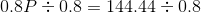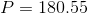, the correct response is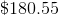### Example Question #24 : How To Find The Sale Price A two-liter bottle of Zippy Zuppy soda costs$1.39. However, as part of a promotion to mark its sixteenth anniversary, if ten or more bottles are purchased, the store is offering a savings of 16%.

Floyd purchases six two-liter bottles of Zippy Zuppy cola, four two-liter bottles of Zippy Zuppy ginger ale, and two two-liter bottles of Zippy Zuppy orange soda. After a sales tax of 6.5%, how much will Floyd pay for the soda (nearest cent)?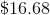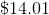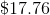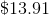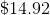Explanation:

Floyd purchases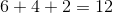bottles of soda, so he will get the discount. Before the discount, the price of the soda is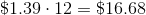The amount of the discount is 16% of this, or (rounding to the nearest cent)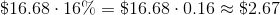making the price before tax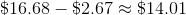.

The sales tax is 6.5% of this, or (again, rounding to the nearest cent)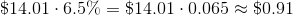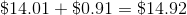,

the amount Floyd pays.

### Example Question #25 : How To Find The Sale Price

The price of a pair of shoes after a 15% clearance discount and a 20% employee discount is $113.74. Give the price of the shoes before the two discounts (nearest cent). Possible Answers: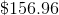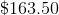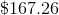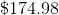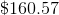Correct answer:Explanation: Call the price of the shoes before the two discounts. A reduction in price by 15%, which is the clearance discount, is equal to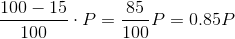A subsequent reduction in price by 20%, which is the employee discount, is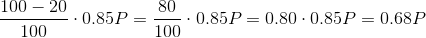Set this quantity equal to the price of the shoes after the two discounts,$113.74, and solve forby dividing by 0.68: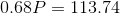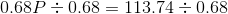To the nearest hundredth: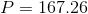The price of the shoes before the discounts was $167.26. ### Example Question #26 : How To Find The Sale Price Katy buys jeans that is on sale for$84. The tag says that the price was originally \$142. What discount is she receiving on the shirt? Round to the nearest tenth of a percent.

None of the other answer options.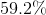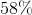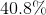Explanation:

Use proportions and cross-multiply to find the percentage of the cost that she is paying, then subtract that answer from the original price to solve for the discount.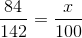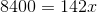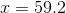If she is paying 59.2% of the regular cost, then subtract that figure from 100%.

100% - 59.2% = 40.8%

You could also set up the proportion so that you could arrive at the answer after cross-multiplying by subtracting 84 from 142 as the first step.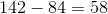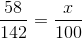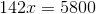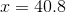1 3 Next →